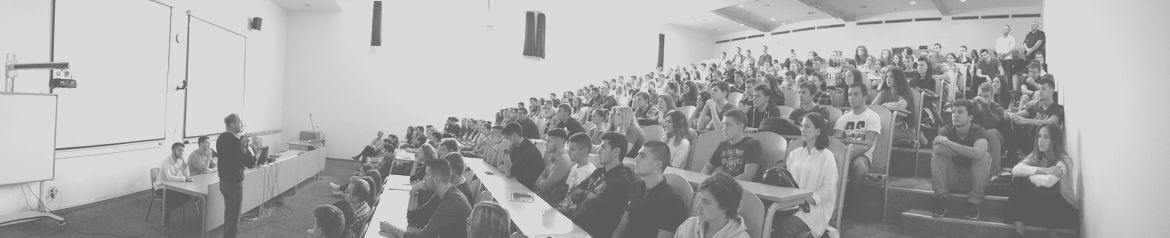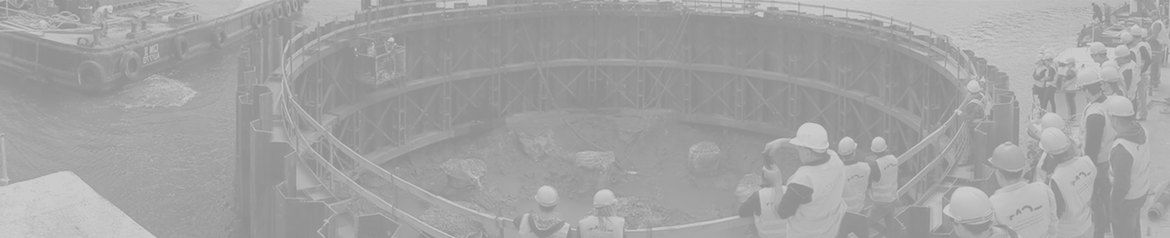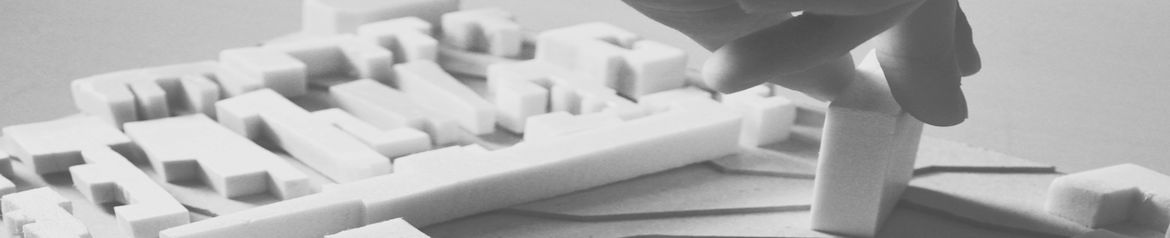•••••# Sveučilište u Splitu - Fakultet građevinarstva, arhitekture i geodezijeUniversity of Split - Faculty of Civil Engineering, Architecture and Geodesy

## Department of Hydromechanics and Hydraulics

 Study Course Semester Hours ECTS Undergraduate University Study of Civil Engineering Hydromechanics IV. 45+45 7,0 Graduate University Study of Civil Engineering Hydraulics I. 45+30 6,0 Undergraduate Professional Study of Civil Engineering Basics of Water Engineering Tunnels and Underground Structures IV. VI. 30+30 30+30 5,0 5,0

Learning outcomes:

Undergraduate University Study of Civil Engineering
Hydromechanics – a student will be able:
- to calculate stationary flow in the pipeline system under the pressure;
- to calculate hydrodynamic force on elbow or fork;
- to calculate the flow under the water-gate;
- to calculate the sharp-edge spillway;
- to calculate uniform and non-uniform flow in open channels with steep and slight slope;
- to define the flow regime in open channels;
- to calculate the piezometric levels of groundwater in aquifer under the pressure and in aquifer with free level surface, for stationary flow;
- to calculate the water in take in the gallery shape.

Graduate University Study of Civil Engineering
Hydraulics
– a student will be able:
- to calculate submerged flow under the water-gate;
- to calculate submerged overflow over the sharp-edged spillway and broad-crested weir;
- to design spillway using WES standard and USBR methodology;
- to calculate and design deepen of stilling basin;
- to calculate stationary flow in water supplay system;
- to calculate non-stationary phenomena in the accumulation system, the supply tunnel and the water chamber;
- to calculate the water hammer in the pipeline with negligible friction, as well as in the friction pipeline,when the valve closes slowly or suddenly;
- to alculate non-stationary phenomena in an pressure system with vessel;
- to calculate seepage below the foundation of the object and to define the vertical forces to the base of the object;
- to analyse the non-stationary water pumping experiment from borehole under the presure.

Undergraduate Professional Study of Civil Engineering
Basics of Water Engineering
- student will be able:
- to calculate stationary flow in system under the pressure with pipes in line;
- to calculate hydrodynamic force on elbow or fork;
- to calculate the flow under the water-gate;
- to calculate the sharp-edge spillway;
- to design spillway using WES standard methodology;
- to calculate uniform flow in open trapezoidal channels;
- to define the flow regime in open channels;
- to calculate the line pressure resistance curve and determine the working point of the pumping plant;
- to calculate the stilling basin;

Tunnels and Underground Structures - student will be able:
- to create rock mass classification: Geomechanical Classification and Classification by Q System;
- to calculate the resistances of the elements of the primary support system;
- to choose the appropriate primary and secondary support system;
- to calculate the total resistance of the selected primary support system;
- to calculate the characteristic curves of the rock mass and the primary support system;
- to calculate stresses in rock mass for elastoplastic rock mass model;
- to be able to lead construction works in the tunnel.